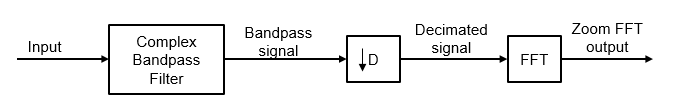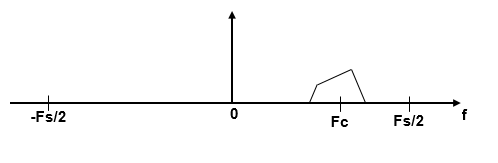# Zoom FFT

High-resolution FFT of a portion of a spectrum

•Libraries:
DSP System Toolbox / Transforms

## Description

The Zoom FFT block computes the fast Fourier Transform (FFT) of a signal over a portion of frequencies in the Nyquist interval. By setting an appropriate decimation factor D, and sampling rate Fs, you can choose the bandwidth of frequencies to analyze BW, where BW = Fs/D. You can also select a specific range of frequencies to analyze in the Nyquist interval by choosing the center frequency of the desired band.

The resolution of a signal is the ratio of Fs and the FFT length (L). Using zoom FFT, you can retain the same resolution you would achieve with a full-size FFT on your original signal by computing a small FFT on a shorter signal. The shorter signal comes from decimating the original signal. The savings come from being able to compute a much shorter FFT while achieving the same resolution. For a decimation factor of D, the new sampling rate, Fsd, is Fs/D, and the new frame size (and FFT length) is Ld = L/D. The resolution of the decimated signal is Fsd/Ld = Fs/L. To achieve a higher resolution of the shorter band, use the original FFT length, L, instead of the decimated FFT length, Ld.

## Ports

### Input

expand all

Input data for which the block computes zoom FFT, specified as a vector or a matrix.

The block treats each column of the input signal as a separate channel. If the input is a two-dimensional signal, the first dimension represents the channel length (or frame size) and the second dimension represents the number of channels. If the input is a one-dimensional signal, then the block interprets it as a single-channel signal.

When you input a variable-size signal (frame length changes during simulation), the frame length of the signal can be arbitrary, that is, the input frame length does not have to be a multiple of the decimation factor. When you input a fixed-size signal (frame length does not change during simulation), the frame length can be arbitrary only when you select the Allow arbitrary frame length for fixed-size input signals parameter.

This port is unnamed until you select the Specify center frequency from input port parameter and click Apply.

Data Types: `single` | `double`
Complex Number Support: Yes

Center frequency of the desired band in Hz, specified as a real scalar in the range (– SampleRate/2, SampleRate/2). SampleRate is either inherited from the input signal or specified through the Input sample rate (Hz) parameter.

#### Dependency

To enable this port, select the Specify center frequency from input port check box and click Apply.

Data Types: `single` | `double`

### Output Arguments

expand all

Zoom FFT output, returned as a vector or matrix.

If you select the Inherit FFT Length from input dimensions check box, the dimensions of the output signal depend on the dimensions of the input signal, the decimation factor of the Zoom FFT block, and whether you select the Allow arbitrary frame length for fixed-size input signals parameter.

This table provides more details on the dimensions of the output signal when you input a fixed-size input signal.

Fixed-Size Input Signal

Input SignalOutput Signal Dimensions

P-by-1 column vector, where P is a multiple of the decimation factor D

(P/D)-by-1

P-by-1 column vector, where P is not a multiple of the decimation factor D

`ceil`(P/D)-by-1 when you select Allow arbitrary frame length for fixed-size input signals.

If you do not select Allow arbitrary frame length for fixed-size input signals, the block errors.

P-by-Q matrix, where P is a multiple of the decimation factor D

(P/D)-by-Q

P-by-Q matrix, where P is not a multiple of the decimation factor D

`ceil`(P/D)-by-Q when you select Allow arbitrary frame length for fixed-size input signals.

If you do not select Allow arbitrary frame length for fixed-size input signals, the block errors.

This table gives more details on the dimensions of the output signal when you input a variable-size input signal. When you input a variable-size signal (frame length changes during simulation), the Allow arbitrary frame length for fixed-size input signals is visible on the block dialog box but does not have any impact on the input frame length. You can input a variable-size signal of any frame length even if you do not select the Allow arbitrary frame length for fixed-size input signals parameter.

Variable-Size Input Signal

Input SignalOutput Signal Dimensions

P-by-Q

`ceil`(P/D)-by-Q

If you clear the Inherit FFT Length from input dimensions check box, the output frame size equals the value you specify in the FFT length(L) parameter.

Input SignalOutput Signal Dimensions

P-by-Q

L-by-Q

The data type of the output is same as the data type of the input.

Data Types: `single` | `double`
Complex Number Support: Yes

## Parameters

expand all

Decimation factor, specified as a positive integer. This value specifies the factor by which the block reduces the bandwidth of the input signal. The number of rows in the input signal must be a multiple of the decimation factor.

Example: `4`

Example: `8`

Data Types: `single` | `double` | `int8` | `int16` | `int32` | `int64` | `uint8` | `uint16` | `uint32` | `uint64`

When you select this option and click , the input port Fc appears on the block icon. You can pass the center frequency through this input port as a scalar.

Center frequency of the desired band in Hz, specified as a real scalar in the range (– SampleRate/2, SampleRate/2). SampleRate is the input sample rate either inherited from the input or specified through the Input sample rate (Hz) parameter.

Example: `0.5`

Example: `10`

#### Dependencies

This parameter applies when you clear the Specify center frequency from input port check box.

Data Types: `single` | `double`

When you select this option, the FFT length is the ratio of the input frame size (number of rows in the input) and the Decimation factor.

FFT length, specified as a positive integer. The FFT length must be greater than or equal to the ratio of the frame size (number of input rows) and the Decimation factor.

Example: `24`

Example: `52`

#### Dependencies

This parameter applies when you clear the Inherit FFT Length from input dimensions check box.

Data Types: `single` | `double` | `int8` | `int16` | `int32` | `int64` | `uint8` | `uint16` | `uint32` | `uint64`

When you clear this check box, the block inherits the sample rate from the input signal.

Input sample rate in Hz, specified as positive real scalar.

Example: `44100`

Example: `48000`

Since R2023a

Specify whether fixed-size input signals (whose size does not change during simulation) can have an arbitrary frame length, where the frame length does not have to be a multiple of the decimation factor. The block uses this parameter only for fixed-size input signals and ignores it if the input has a variable-size.

When the input signal is a variable-size signal, the signal can have arbitrary frame length, that is, the frame length does not have to be a multiple of the decimation factor.

For fixed-size input signals, if you:

• Select the Allow arbitrary frame length for fixed-size input signals parameter, the frame length of the signal does not have to be a multiple of the decimation factor. If the input is not a multiple of the decimation factor, then the output is generally a variable-size signal. Therefore, to support arbitrary input size, the block must also support variable-size operations, which you can enable by selecting the Allow arbitrary frame length for fixed-size input signals parameter.

• Clear the Allow arbitrary frame length for fixed-size input signals parameter, the input frame length must be a multiple of the decimation factor.

Type of simulation to run. You can set this parameter to:

• `Interpreted execution`: Simulate model using the MATLAB® interpreter. This option shortens startup time.

• `Code generation`: Simulate model using generated C code. The first time you run a simulation, Simulink generates C code for the block. The C code is reused for subsequent simulations as long as the model does not change. This option requires additional startup time but provides faster subsequent simulations.

## Block Characteristics

 Data Types `double` | `single` Multidimensional Signals `No` Variable-Size Signals `Yes`

## Algorithms

The zoom FFT algorithm leverages bandpass filtering before computing the FFT of the signal. The concept of bandpass filtering is that suppose you are interested in the band [F1, F2] of the original input signal, sampled at the rate Fs Hz. If you pass this signal through a complex (one-sided) bandpass filter centered at Fc = (F1+F2)/2, with the bandwidth BW = F2F1, and then downsample the signal by a factor of D = floor(Fs/BW), the desired band comes down to the baseband.If Fc cannot be expressed in the form of k×Fs/D, where k is an integer, then the shifted, decimated spectrum is not centered at DC. In this case, the center frequency gets translated to Fd.

`${F}_{d}={F}_{c}-\left({F}_{s}/D\right)×floor\left(\left(D×{F}_{c}+{F}_{s}/2\right)/{F}_{s}\right)$`

The complex bandpass filter is obtained by first designing a lowpass filter prototype and then multiplying the lowpass coefficients with a complex exponential. This algorithm uses a multirate, multistage FIR filter as the lowpass filter prototype. To obtain the bandpass filter, the coefficients of each stage are frequency shifted. The decimation factor is the cumulative decimation factor of each stage. The complex bandpass filter followed by the decimator are implemented using an efficient polyphase structure. For more details on the design of the complex bandpass filter from the multirate multistage FIR filter prototype, see Zoom FFT and Complex Bandpass Filter Design.

 Harris, F.J. Multirate Signal Processing for Communication Systems. Prentice Hall, 2004, pp. 208–209.